# Teaching

These are course notes, homeworks, quizzes, exams from old and current courses. Not for every course. And not every exam or quiz or HW.

### Current and Recent Courses

Algebraic Geometry-I

Abelian Varieties

Rigid Analytic Geometry (Aug-Nov 2019)

### Older Courses

Differential Equations (Jan-April 2019)

Introduction to Combinatorics (U of Toronto, Winter 2018)

Compact Riemann Surfaces (Jan-April 2013)

Torsors (Aug-Nov 2012)

Sheaves and Cohomology (Aug-Nov 2010)

Nurture Lectures (Summer 1998)

##### MGE023 Algebraic Geometry-I (Sep-Dec 2021) (Current)

The course will cover basic scheme theory, cohomology of quasi-coherent sheaves, Serre duality for proper schemes, Riemann-Roch for curves and surfaces, and applications of these techniques.

The desingularisation of a lemniscate obtained by looking at the proper transfrom of the lemniscate by blowing up the plane at the singularity. The red curve is the proper transform of the lemniscate. The z-axis is the exceptional divisor.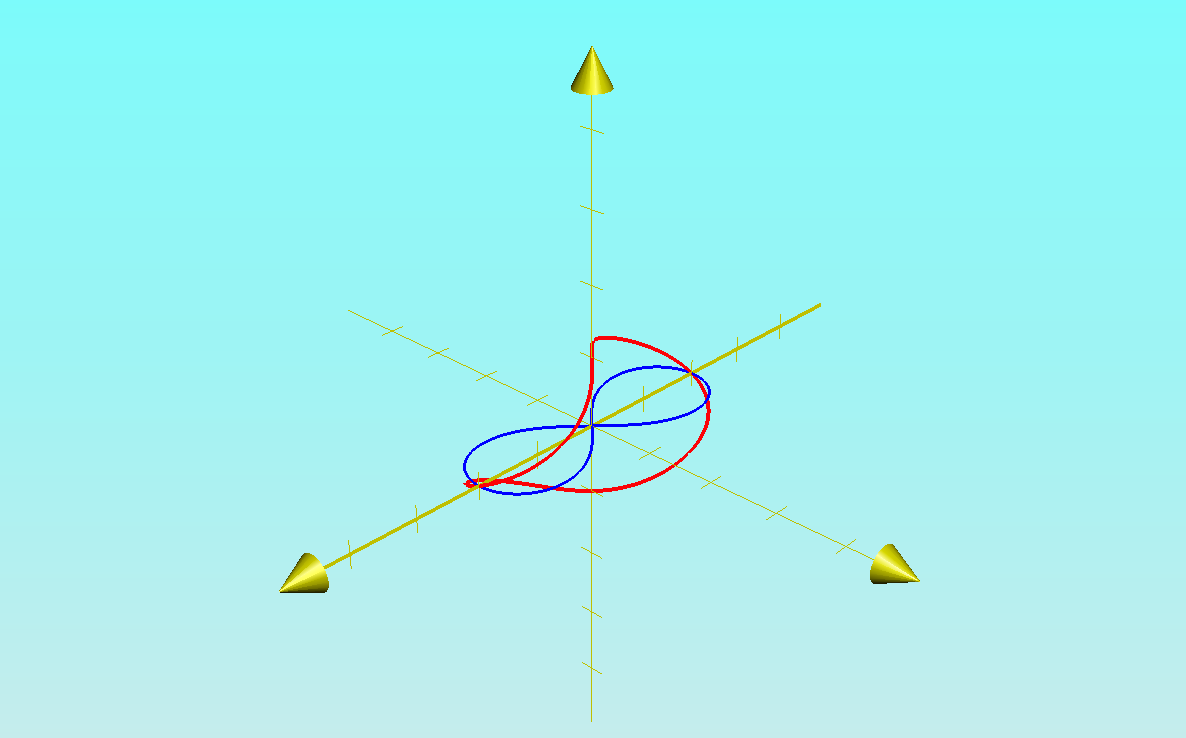##### MGE050 Abelian Varieties (Jan-April 2021)

The course covered the basic theory of abelian varieties via scheme theory. Topics included: Embedding of abelian varieties in projective space, Isogenies, Riemann-Roch for abelian varieties, the index of a line bundle, criterion for ampleness of a line bundle via its Hilbert polynomial, the construction of the dual abelian variety of a given abelian variety.

The picture of an abelian variety of dimension one over the complex numbers##### MU2202 Differential Equations

The course covers basic techniques of solving ordinary differential equations (separable, linear with constant coefficients, variation of parameters, etc) and theorems on the existence, uniquenss of solutions, their variation with initial conditions and parameters, rectification of vector fields, one-parameter groups of diffeomorphisms and the associated autonomous differential equations, the equation of variations associated to differential equation whose vector field is C1, linearisation, and more.

Here is a picture of an integral curve of the logistic equation y'=y(1-y) when the phase space is compactified by a one point compactification of the y-axis. The compactification is the red circle.The blue curve is an integral curve whose initial phase is greater than 1, the thin black line on the right is the line y=∞, the red line the usual time axis y=0, and (faint) the green line on top the line y=1. The blue dot is where the inegral curve crosses y=∞. See Lectures 17 and 18 below.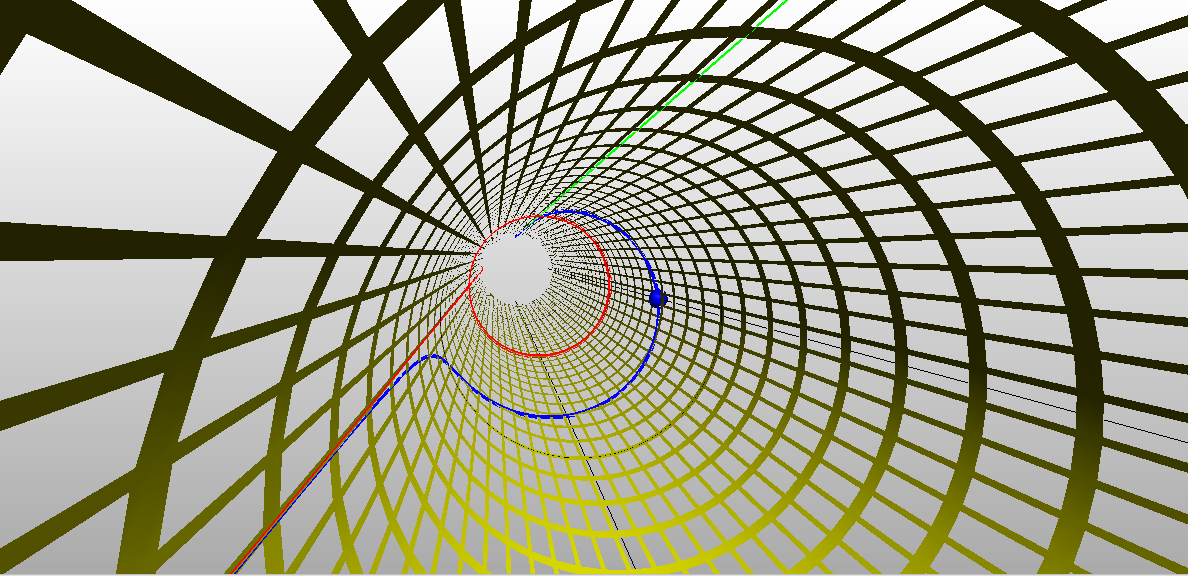Topology of Rn: Euclidean, l1 and l norms on Rn and the equivalence of convergence of sequences in Rn, open and closed sets, sequential compactness, continuous functions defined on subsets of Rn, boundedness of continuous functions defined on compact sets. Characterisation of open sets in Rn.

Differentiation: Review of inner product spaces and linear maps on inner product spaces, Derivative as a linear map, Chain rule, Matrix representation and and partial derivatives, Comparison of real and complex derivatives, sufficient condition for differentiability, equality of mixed partial derivatives. Taylor's formula and its application to maxima and minima, Inverse function theorem, Implicit function theorem, Tangent space of level sets and gradient, Lagrange multiplier method, diagonalisation of symmetric operators.

Here is a picture of the surface x2+y3 -y2+z2=0 used in Lecture 10 below to illustrate the Implicit Function Theorem.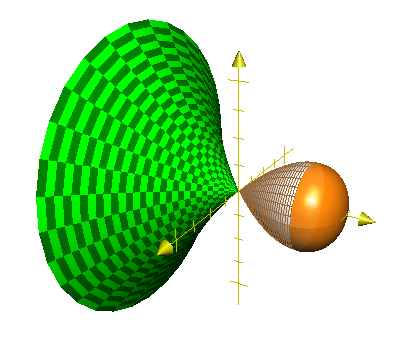##### MGE094 Rigid Analytic Geometry (Aug-Nov 2019 )

Non-archimedean fields, Tate algebras, affinoid algebras, rigid spaces, GAGA for rigid spaces, Tate's uniformisation of elliptic curves, Mumford's uniformisation theorem.

Here is how ultra-metrics can be visualisd. This is Figure 2 in Jan E. Holly's very readable article Pictures of Ultrametric Spaces, the p-adic Numbers, and Valued Fields, American Mathematical Monthly, October 2001.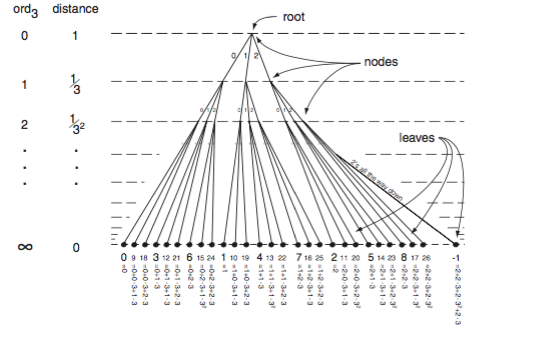##### MU2202 Differential Equations (Jan-April 2019)

Please see the current course (i.e. Jan-April 2021) on Differential Equations for files. The files for the 2019 course are no longer available.

##### Graduate Analysis I (Aug-Nov 2018)

Measure Theory, basic functional analysis, Lp spaces, Fourier series, Fourier integrals, Fourier inversion formula.

And here are some approximate identities:##### Introduction to Combinatorics MAT344 (Winter 2018 at U of Toronto)

The graph below is crucial to computing Catalan numbers. See Lecture 23.##### Graduate Complex Analysis (Jan-April 2017)

Picture of Schwarz reflections of triangles in the unit disc leading to a proof of the Little Picard Theorem. See Lecture 26 for details.##### Graduate Algebra I (Aug-Nov 2015)

The Notes are divided by topics rather than by lectures. Not everything taught is up on the website.

Below is a picture ocurring in the proof of the Snake Lemma in an abstract abelian category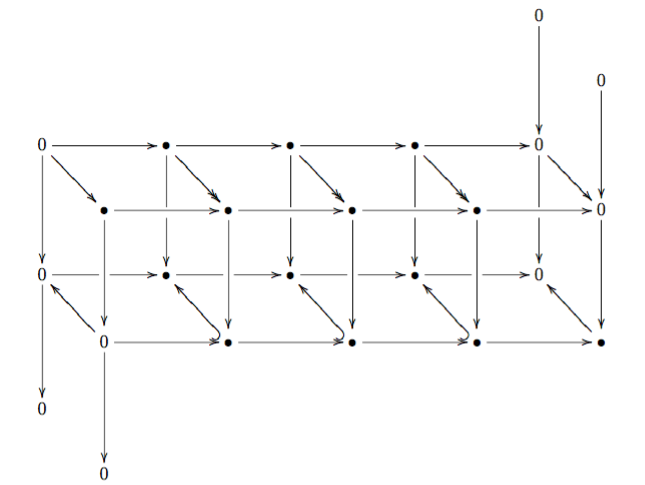##### Compact Riemann Surfaces (Jan-April 2013)

The course revolved around Homework problems (see the outline), and I haven't scanned my lecture notes for the subject. The HW problems may be of some interest.

##### Torsors (Aug-Nov 2012)

The course covered the basics of torsors, regarding them as algebraic geometric analogues of principal bundles. The first few lectures covered the very basics of principal bundles on manifolds. Later the course covered faithful flat descent, fpqc, fpff topologies, schemes as sheaves in the fpqc topology, torsors in this setting, reduction of structure groups in this general setting, as well as fibre bundles associated with a torsor and the action of the underlying group scheme on a scheme.